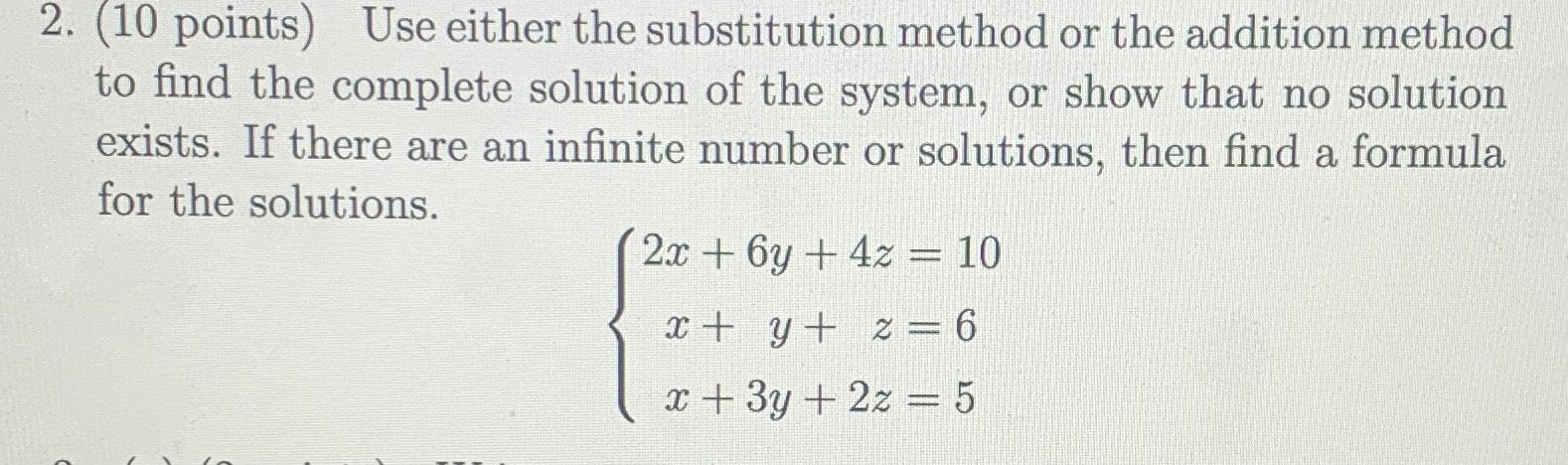### ¿Todavía tienes preguntas de matemáticas?

Pregunte a nuestros tutores expertos
Algebra
Pregunta2. (10 points) Use either the substitution method or the addition method to find the complete solution of the system, or show that no solution exists. If there are an infinite number or solutions, then find a formula for the solutions.

$$\left\{ \begin{array} { l } { 2 x + 6 y + 4 z = 10 } \\ { x + y + z = 6 } \\ { x + 3 y + 2 z = 5 } \end{array} \right.$$

x=$$\frac{13}{2} - \frac{z}{2}$$
y=$$- \frac{1}{2} - \frac{1}{2} z$$